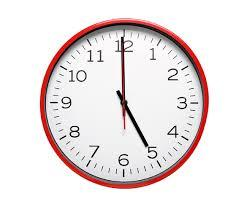# Simultaneously 3157

The first machine closes 65 closing bottles per minute. The second machine closes five bottles less at the same time. How many minutes will both machines close 2,500 bottles if they work simultaneously?

x =  20 min

### Step-by-step explanation:

65x+(65-5)x = 2500

65·x+(65-5)·x = 2500

125x = 2500

x = 2500/125 = 20

x = 20

Our simple equation calculator calculates it.Did you find an error or inaccuracy? Feel free to write us. Thank you!

Tips for related online calculators
Do you have a linear equation or system of equations and looking for its solution? Or do you have a quadratic equation?
Do you want to convert time units like minutes to seconds?